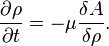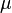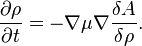# Dynamical density-functional theory

Dynamical Density functional theory is a set of theories in statistical mechanics that extends equilibrium density-functional theory to situations away from equilibrium. In the simplest case, only small deviations from equilibrium are considered, so that linear response theory can be applied.

A simple approach in this line is to consider this evolution of the density field:$\frac{\partial\rho}{\partial t}= - \mu \frac{\delta A}{\delta\rho}.$

In equilibrium, the left hand side vanishes and one is left with the usual density functional expression. Away from it, an increase in the Helmholtz energy function,$A$, causes a decrease in the density, mediated by the mobility coefficient$\mu$.

This sort of evolution will not satisfy conservation of the number of particles (that is, the space integral of the density field), and is therefore termed non-conserved dynamics. This can be valid in cases in which this field is in fact not conserved, such as the magnetisation field in a model for magnets (such as the Ising model).

In other cases, for example with actual particles, some evolution has to be postulated. For example, this evolution will conserve the number of particles:$\frac{\partial\rho}{\partial t}= - \nabla \mu \nabla \frac{\delta A}{\delta\rho}.$

The later sort of expressions are called non-conserved dynamics.